# Wilcoxon Signed Rank Test: Definition, How to Run, SPSS

Share on

Contents:

## What is the Wilcoxon Signed Rank Test?

The Wilcoxon signed rank test (also called the Wilcoxon signed rank sum test) is a non-parametric test to compare data. When the word “non-parametric” is used in stats, it doesn’t quite mean that you know nothing about the population. It usually means that you know the population data does not have a normal distribution. The Wilcoxon signed rank test should be used if the differences between pairs of data are non-normally distributed.

Watch the video for an overview and how to run the Wilcoxon signed rank test by hand:

Two slightly different versions of the test exist:

• The Wilcoxon signed rank test compares your sample median against a hypothetical median.
• The Wilcoxon matched-pairs signed rank test computes the difference between each set of matched pairs, then follows the same procedure as the signed rank test to compare the sample against some median.

The term “Wilcoxon” is often used for either test. This usually isn’t confusing, as it should be obvious if the data is matched, or not matched.

The null hypothesis for this test is that the medians of two samples are equal. It is generally used:

## How to Run the Wilcoxon Signed Rank Test in SPSS

Watch the video to learn how to run the Wilcoxon test in SPSS:

## Other Technology

R: You can find sample code here on RCompanion.org.

## How to Run the Test by Hand

Need help with the formulas? Check out our tutoring page!

Requirements for running the matched pairs test:

• Data must be matched.
• The dependent variable must be continuous (i.e. you must be able to distinguish between values at the nth decimal place).
• You should have no tied ranks for maximum accuracy. If ranks are tied, there is a workaround (see below, after Step 5).

Example question: Is there a difference between the median values for the following sets of treatment data for the twelve groups?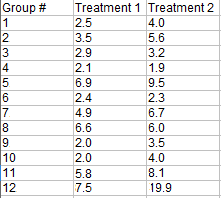Step 1: Subtract treatment 2 from treatment 1 to get the differences: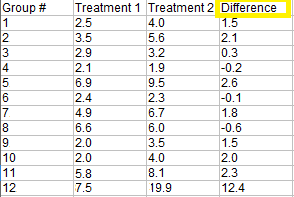Note: If you only have one sample, calculate the differences between each variable and zero (the hypothesized median) instead of the difference between pairs.

Step 2: Place the differences in order (column 2 in the picture below), and then rank them. Ignore the sign when placing in rank order.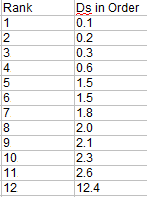Step 3: Make a third column and note the sign of the difference (the one you ignored in Step 2!).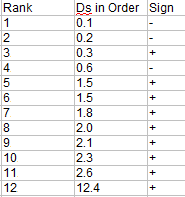The next two steps calculate the Wilcoxon signed rank sums:

Step 4: Calculate the sum of the ranks of the negative differences (the ones with the negative sign in the Step 3 chart). You’re adding up the ranks here, not the actual differences:
W-- = 1 + 2 + 4 = 7

Step 5: Calculate the sum of the ranks of the positive differences (the ones with the positive sign in the Step 3 chart).
W+ = 3 + 5.5 + 5.5 + 7 + 8 + 9 + 10 + 11 + 12 = 71

## Wilcoxon Signed Ranks Test Statistic and Critical Value

1. Use the smaller of W+ or W-- for the test statistic. For this example, Wstat = 7.
2. Compare your test statistic to a critical value (see table below). For this example, our critical value for N = 12 and a two-tailed test (because no direction is specified) is 13 (at a 5% alpha level).

Our test statistic (7) is smaller than the critical value (13), so there is a significant difference in the medians. We can reject the null hypothesis.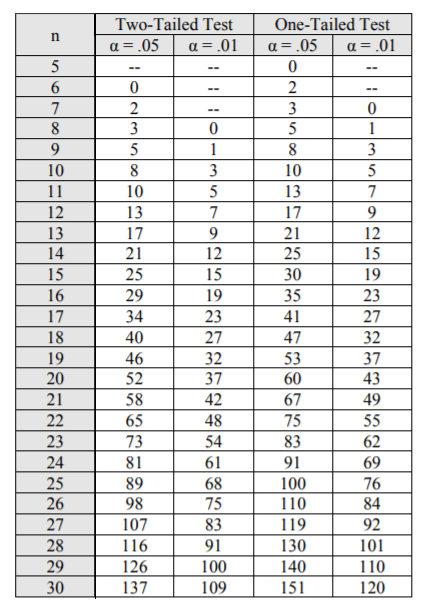## References

Table: http://users.stat.ufl.edu/~winner/tables/wilcox_signrank.pdf
Breslow, N. (1970) A generalized Kruskal-Wallis test for comparing K samples subject to unequal patterns of censorship, Biometrika, 57(3), 579–594.
Gonick, L. (1993). The Cartoon Guide to Statistics. HarperPerennial.
Kotz, S.; et al., eds. (2006), Encyclopedia of Statistical Sciences, Wiley.
Everitt, B. S.; Skrondal, A. (2010), The Cambridge Dictionary of Statistics, Cambridge University Press.
Vogt, W.P. (2005). Dictionary of Statistics & Methodology: A Nontechnical Guide for the Social Sciences. SAGE.

CITE THIS AS:
Stephanie Glen. "Wilcoxon Signed Rank Test: Definition, How to Run, SPSS" From StatisticsHowTo.com: Elementary Statistics for the rest of us! https://www.statisticshowto.com/probability-and-statistics/statistics-definitions/wilcoxon-signed-rank-test/
---------------------------------------------------------------------------Need help with a homework or test question? With Chegg Study, you can get step-by-step solutions to your questions from an expert in the field. Your first 30 minutes with a Chegg tutor is free!